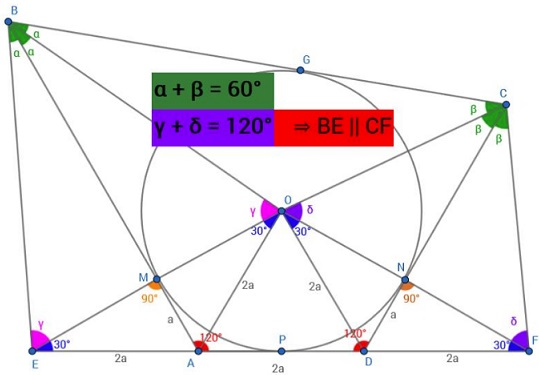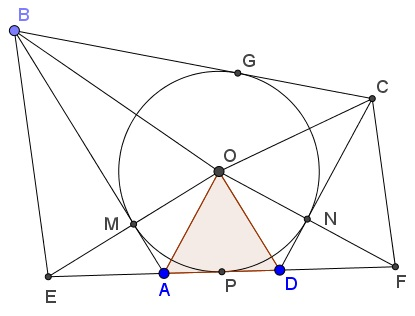# A Problem in a Special Tangential Quadrilateral

### Source### Solution### A few words

Quadrilateral $ABCD$ being tangential, there is a circle tangent to all four sides of the quadrilateral. Let $O$ be its center and $M,$ $P,$ $N,$ $G$ the points of tangency, as depicted below:Note that $AO,$ $BO,$ $CO,$ $DO$ are the bisectors of the angles at $A,$ $B,$ $C,$ $D,$ respectively. Since the angles at $A$ and $D$ are $120^{\circ},$ $\Delta ADO$ is equilateral.

Extend $AD$ both directions to $E$ and $F$ so that $AE=AD=DF.$ Then, e.g., $\Delta AEO$ is isosceles and $AM$ is the bisector of $\angle EAO$ and the median to $AO:$ $AM=MO.$ In addition, $AM\perp AO,$ i.e., $AB\perp EO,$ implying $\Delta EBO$ is isosceles and making $\angle EBM=\angle MBO.$ But, since both $BM$ and $BG$ are tangent to $(O),$ $\angle MBO=\angle OBG.$ Thus lines $BA$ and $BO$ trisect $\angle EBC.$

Similarly, lines $CO$ and $CD$ trisect $\angle BCF.$

Now, by pure angle chasing,

$\angle AEO=\angle AOE=30^{\circ},\\ \angle BEO=\angle BOE=90^{\circ}-\displaystyle \frac{1}{2}\angle ABC,\\ \angle OFD=\angle DOF=30^{\circ},\\ \angle CFO=\angle COF=90^{\circ}-\displaystyle \frac{1}{2}\angle BCD.$

It follows that

\begin{align}\angle BEF+\angle CFE &= (\angle AEO+\angle BEO)+(\angle OFD+\angle CFO)\\ &=30^{\circ}+90^{\circ}-\displaystyle \frac{1}{2}\angle ABC+30^{\circ}+90^{\circ}-\displaystyle \frac{1}{2}\angle BCD\\ &=240^{\circ}-\displaystyle \frac{1}{2}(\angle ABC+\angle BCD)\\ &=240^{\circ}-60^{\circ}=180^{\circ} \end{align}

Because $\angle ABC+\angle BCD=360^{\circ}-120^{\circ}-120^{\circ}=120^{\circ}.$

Thus lines $BE$ and $CF$ are parallel so that the lines parallel to the two through $A$ and $D$ divide $BC$ into equal segments, and vice versa.

### Acknowledgment

The problem has been proposed by Thanos Kalogerakis at the Peru Geometrico facebook group. Thanos then kindly informed me of his post and suggested that the problem was related to a variant of Morley's theorem that was published years ago. The link notwithstanding, I was beaten to it by Daniel Dan, whose graphical solution appeared above.

Thanos further proposed that $\displaystyle \frac{1}{AB}+\frac{1}{CD}=\frac{1}{AD}.$ This is a disguised content of his other problem.